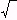edHelper subscribers - Create a new printable

Sample edHelper.com - Functions Worksheet

 Name _____________________________Date ___________________
Relations and Functions
Identify the transformation from f(x) to g(x).

1.
f(x)  =
 x 2 - 4
g(x)  =
 ( 8 x ) 2 - 4

2.
f(x)  =( 4 x )
g(x)  =
 49( 4 x )

3.
f(x)  =
 ( x - 4 ) 2
g(x)  =
(
 17
x  -  4)2

4.
f(x)  =
|
 -2 x
|   -  4
g(x)  =
2 |
 -2 x
|   -  4

5.
f(x)  =
|
 x - 3
|
g(x)  =
|
 6 x - 3
|

6.
f(x)  =
 x 2
g(x)  =
 ( x + 3 ) 2 - 3

7.
f(x)  =
 x 2
g(x)  =
 - ( x - 9 ) 2

8.
f(x)  =
4( x + 3 )
g(x)  =
-4(
 16
x  +  3)

9.
f(x)  =
4( x )
g(x)  =
-4(
 13
x)
-  7

10.
f(x)  =
 ( x ) 2
g(x)  =
 3 ( x + 7 ) 2

11.
f(x)  =
 3 x 2 - 2
g(x)  =
 -3 ( 4 x ) 2 - 2

12.
f(x)  =
3 |
 x
|
g(x)  =
 32
|
 8 x
|

13.
f(x)  =
 x 2
g(x)  =
2(
 -15
x)2  +  6

14.
f(x)  =( x )
g(x)  =(
 18
x)
-  7

Sample
This is only a sample worksheet.

edHelper subscribers - Create a new printable